• Introduction
• What is in this manual
• What is Caspoc
• User interface
• Introduction
• Starting
• Simulation
• Editing
• Viewing and printing
• Getting Started
• Basic editing
• Simulation in the time domain
• Basic User Interface Topics
• Editing
• Simulation
• Viewing
• Library
• Reports
• Project management
• Circuit and Block Diagram Components
• Introduction
• Cscript and user defined functions
• Component parameters
• Modeling Topics
• Introduction
• Power Electronics
• Semiconductors
• Electrical Machines
• Electrical drives
• Power Systems
• Mechanical Systems
• Thermal Systems
• Magnetic Circuits
• Green Energy
• Coupling to FEM
• Experimenter
• Analog hardware description language
• Embedded C code Export
• Coupling to Spice
• Small Signal Analysis
• Matlab coupling
• Tips and tricks
• Appendices

In this tutorial we will explore the animation feature that can help you understand electric circuits. We will create a resistive circuit and Ohm's law will be the topic for the animation. An electric circuit will be constructed as shown below. Select the circuit components from Components/Circuit/Sources and Components/Circuit/RLCDuring the animation the voltage at each circuit node will be displayed as long as a label is defined at the node. Since we want to see all the voltages in the electric circuit, we define the label A and B in the electric circuit as shown below. The labels are defined by clicking the node with the right mouse button and type the label in the input field of the label dialog box.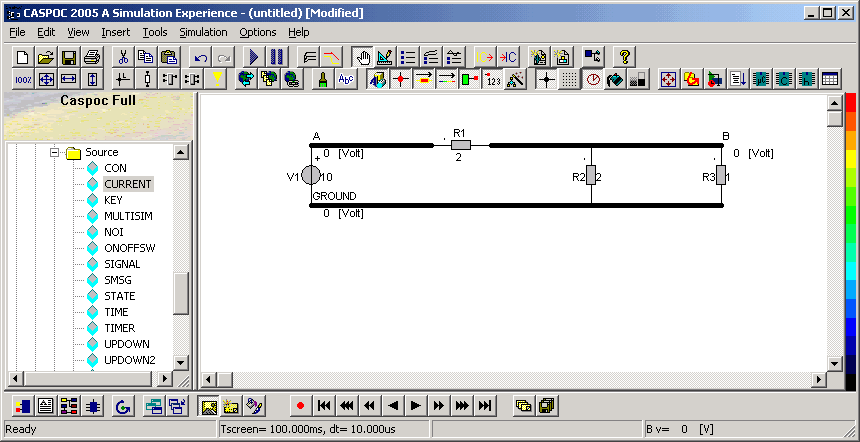We also want to know the current through all components, so we place 4 CURRENT blocks on the work-screen. Select the circuit components from Components/Blocks/Source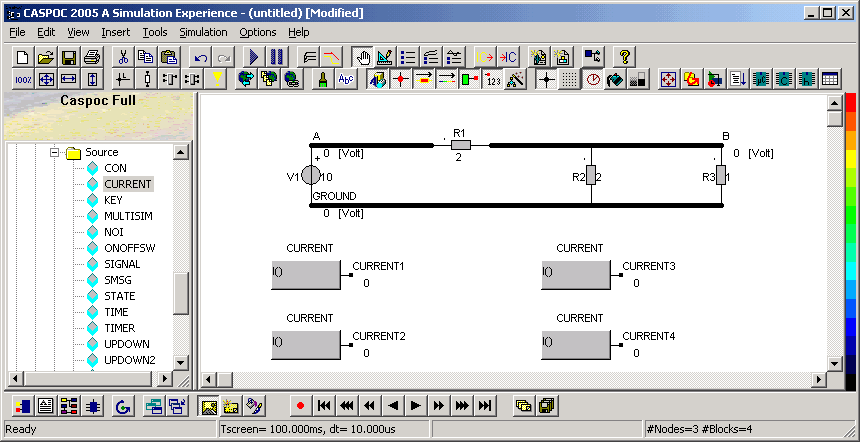We want to measure the current through each component. Therefore we define at each CURRENT block the name of a circuit component, as done in the previous tutorial on the multilevel simulation. Open each CURRENT block by clicking it with the rigth mouse button. At the text input field enter the name of the circuit component where you want to measure the current through.During the animation, the current through the wires is indicated by a color as well as the level of the voltage. We first have to set the animation parameters. Do this by opening the animation parameters dialog box by selecting Options/Animation parameters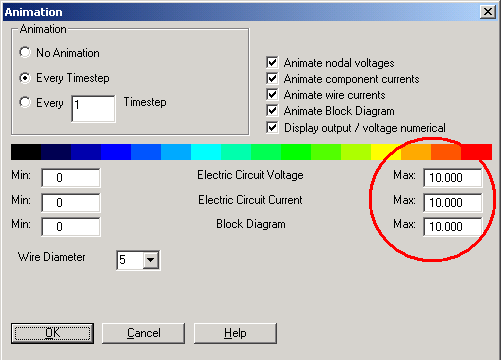You can set the width of the wire in the electric circuit by changing the Wire Diameter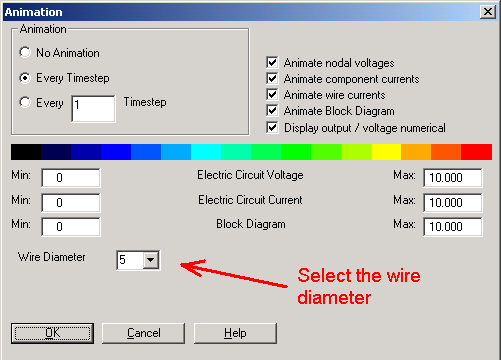Before we start the animation, be sure that all animation options are enabled. Simply take care that all buttons are enabled as shown in the following button bar.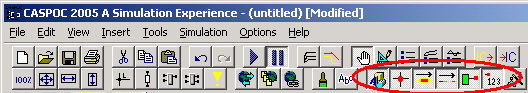Start the simulation by pressing the Play buttonor pressing the [Enter] key. The simulation is now started and the calulated voltages on each node and the current through the components are shown.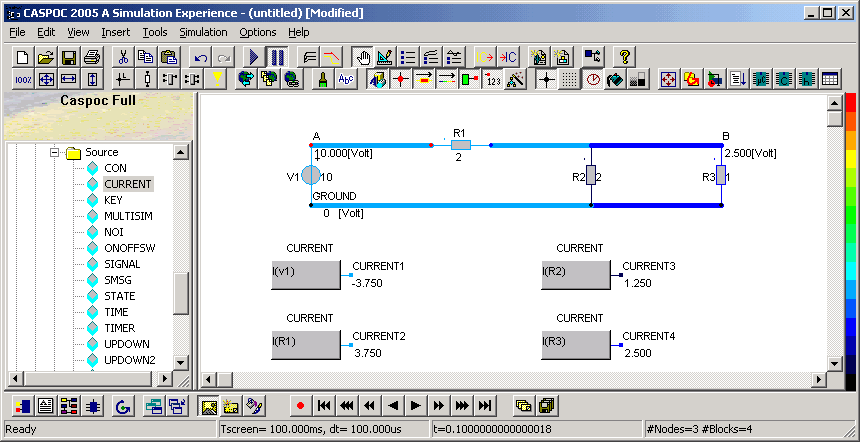The animation shows all nodal voltages and the currents through the components. Note that the current flows into a component following the dot convention. The current through a voltage source is positive if it flows from the positive node through the voltage source to the negative node. Therefore the measured current through our voltage source is indicated to be negative.